# Proof of Wolfram's Axiom for Boolean Algebra

Steps in an automated proof of the correctness of Wolfram’s Axiom

The goal is to prove as theorems the three standard Sheffer axioms of Boolean algebra from Wolfram's single axiom.

Each step states what is being proved, then gives a list of the steps required for a proof.

The result is a proof that Wolfram's Axiom is a complete axiom for Boolean algebra. It is the simplest possible one.

The proof was first given in very small type on pages 810 and 811 of "A New Kind of Science".

Each individual step in the proof is performed by inserting the specified axiom or lemma, essentially using pattern matching, but with substitutions and rearrangements for variables being made.

(2 columns, 85 rows)

## Examples

### Basic Examples

#### Retrieve the resource:

 In:=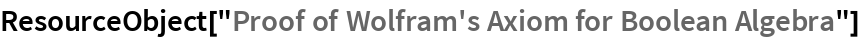Out=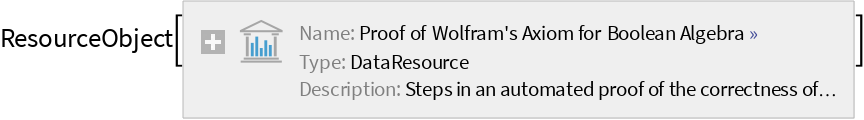#### Show the 20th step:

 In:=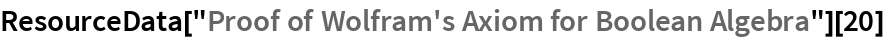Out=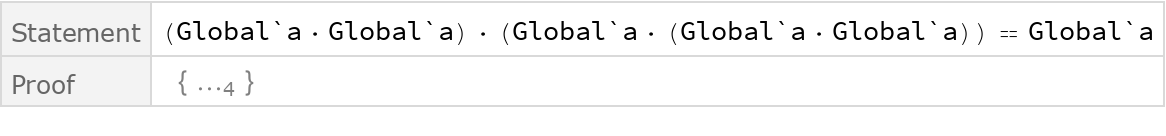#### Show the corresponding proof:

 In:=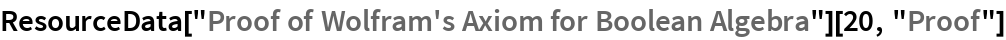Out=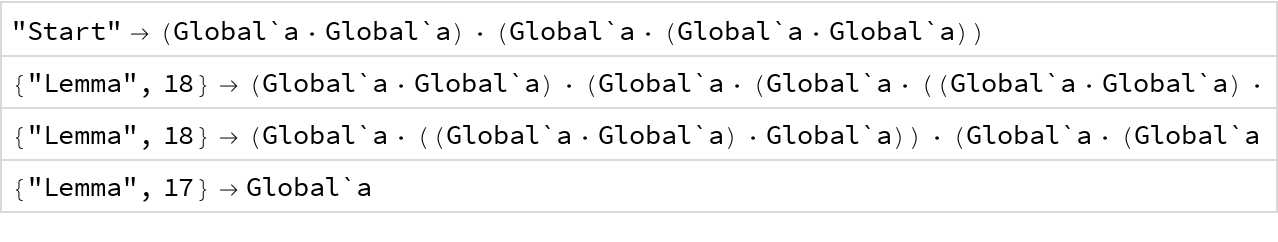#### Show the data associated with lemma 20:

 In:=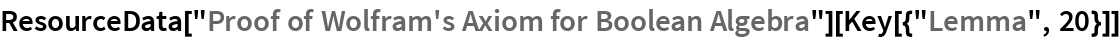Out=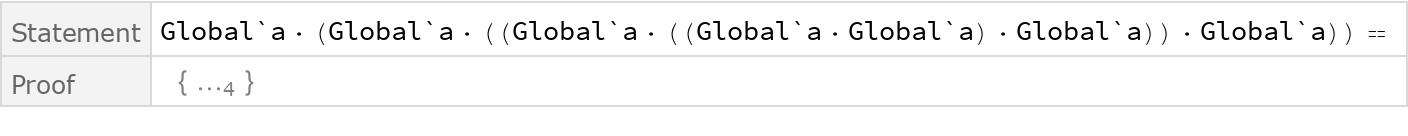#### Find the number of lemmas used in the proof:

 In:=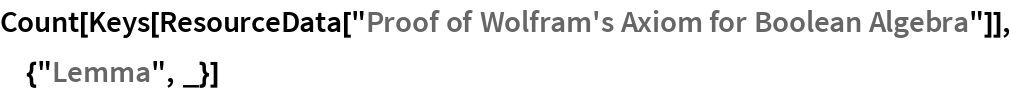Out=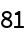#### Pick out the statements used on the first 5 steps:

 In:=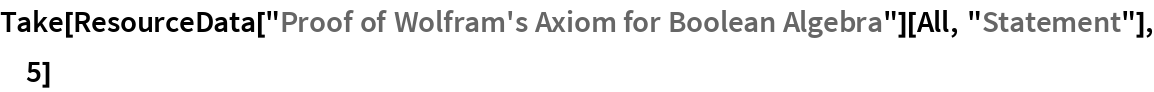Out=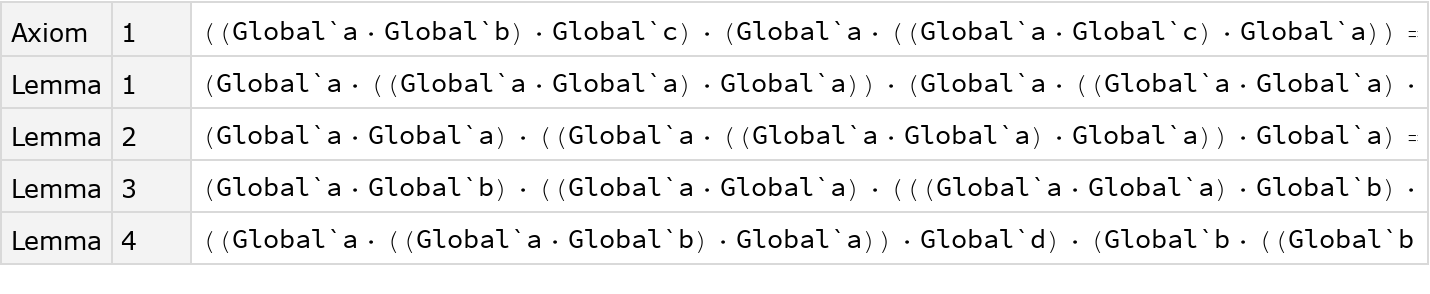#### Plot the lengths of the statements used on all steps:

 In:=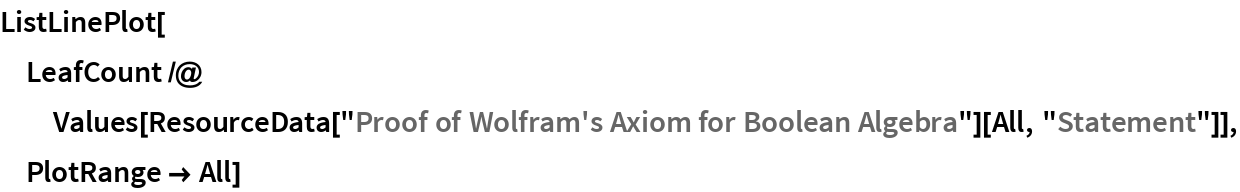Out=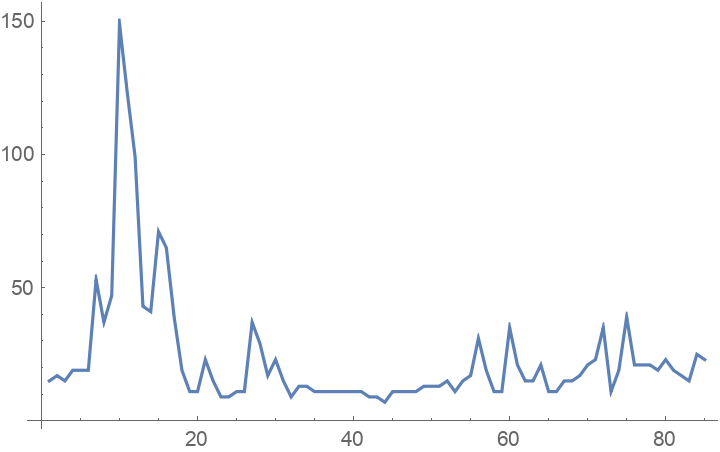#### Find the longest statement:

 In:=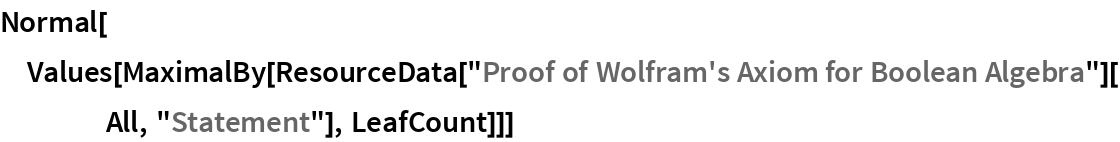Out=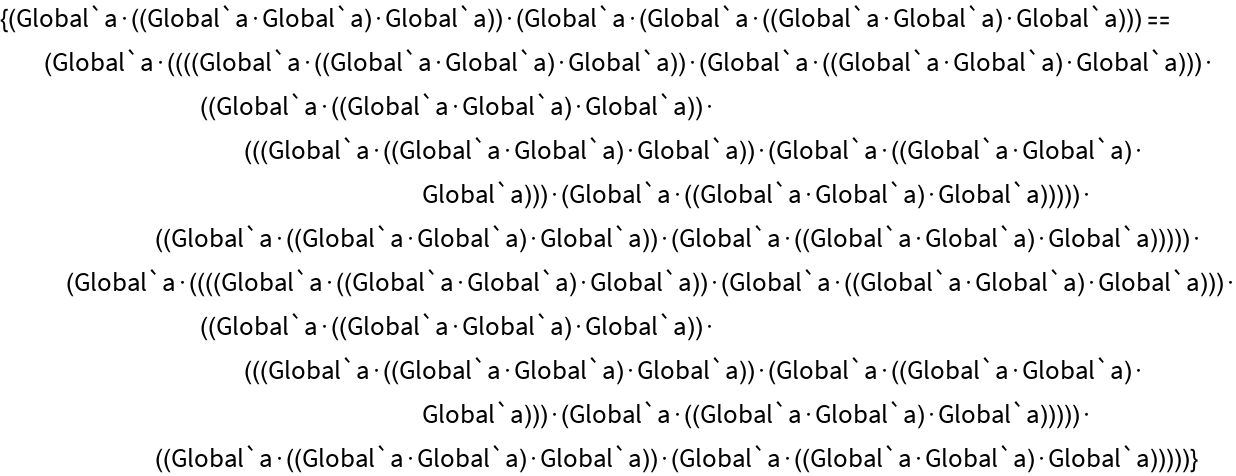#### Show the statement as a tree:

 In:=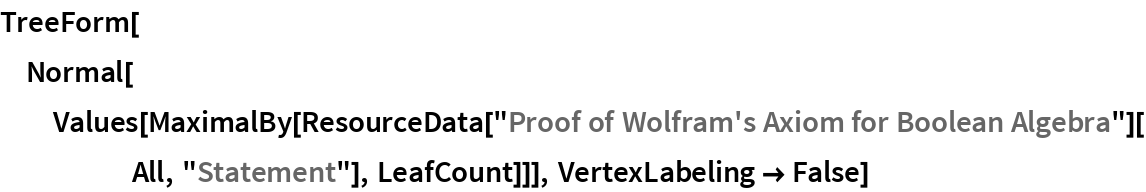Out=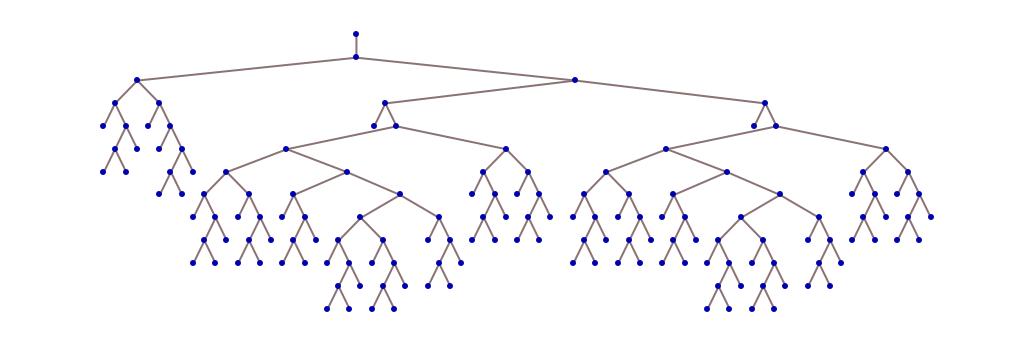In:=#### Plot the lengths of the proofs used:

 In:=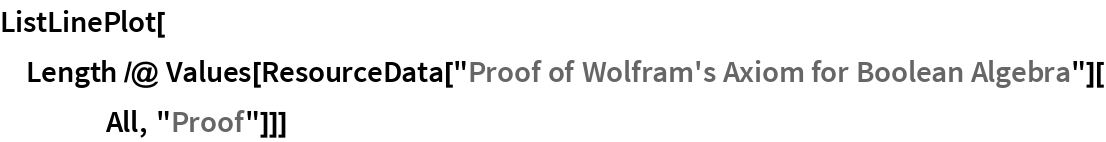Out=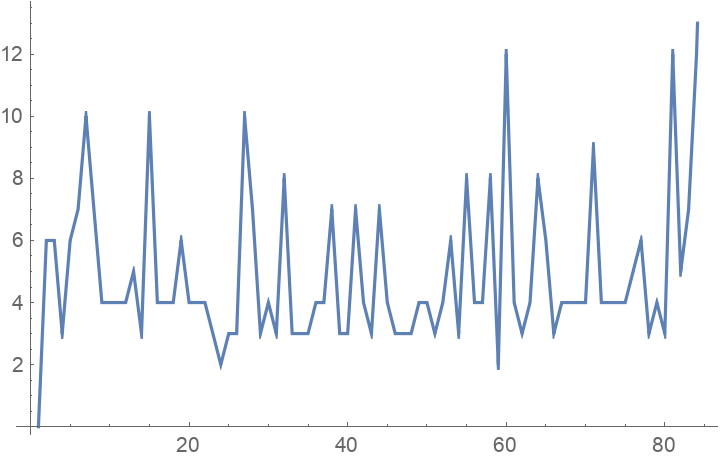#### Find the chain of dependencies in the first 5 steps:

 In:=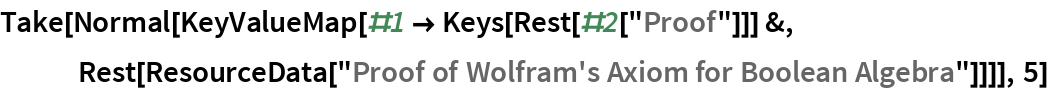Out=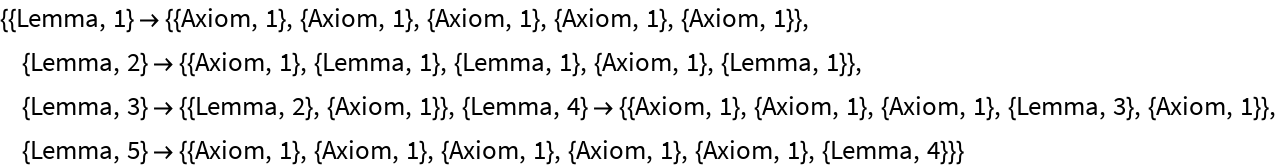#### Make a graph of dependencies:

 In:=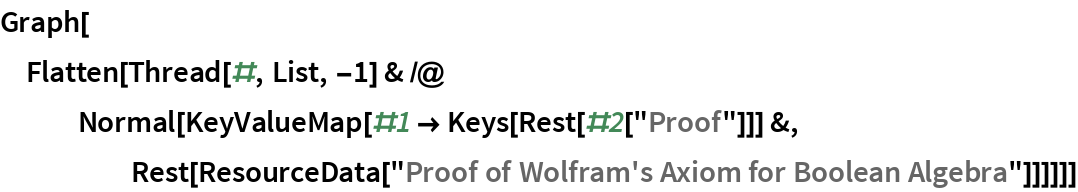Out=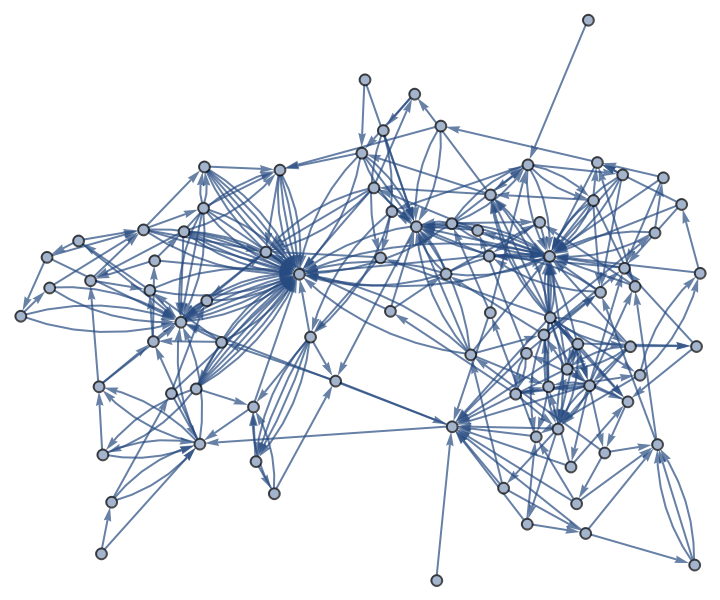#### A layered version:

 In:=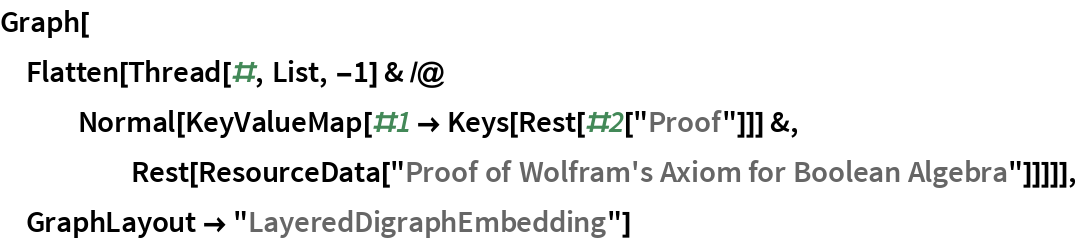Out=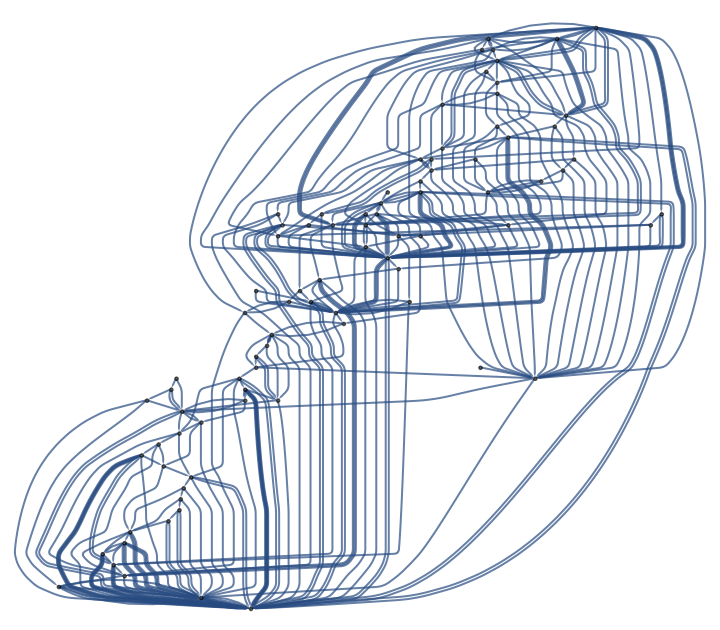#### A linear version:

 In:=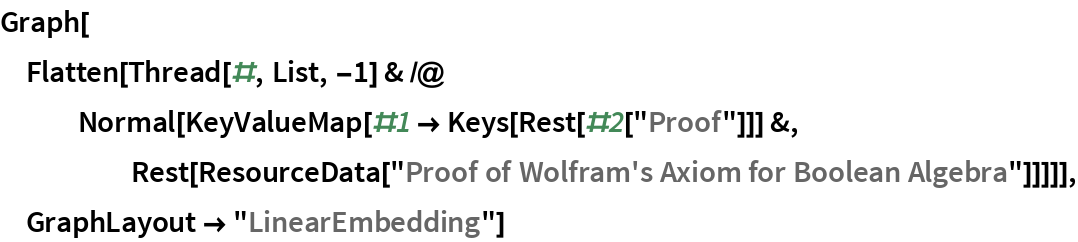Out=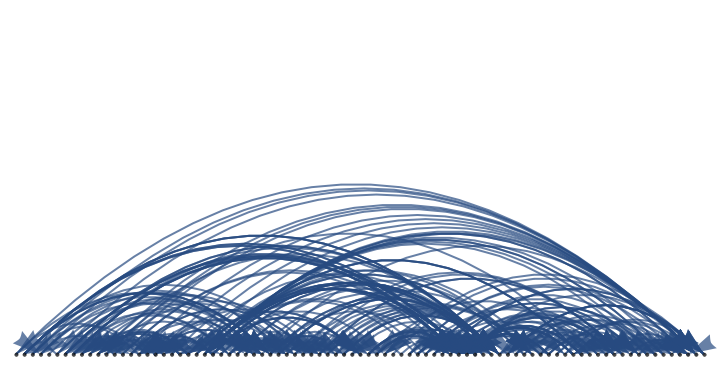#### Find the original axiom:

 In:=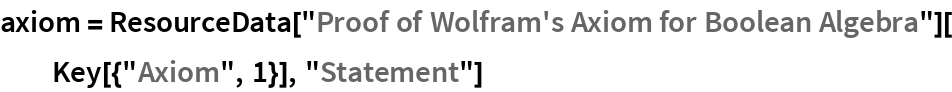Out=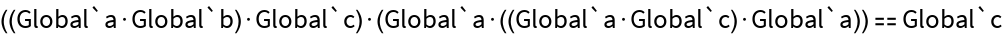#### Find the statement of Lemma 1:

 In:=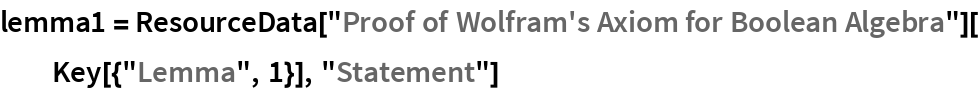Out=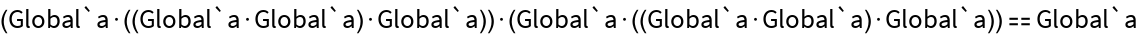#### Determine that Lemma 1 can be proved from the axiom:

 In:=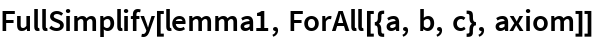Out=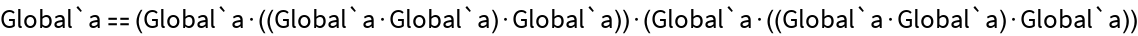#### Show the succession of trees used in the proof of Lemma 1:

 In:=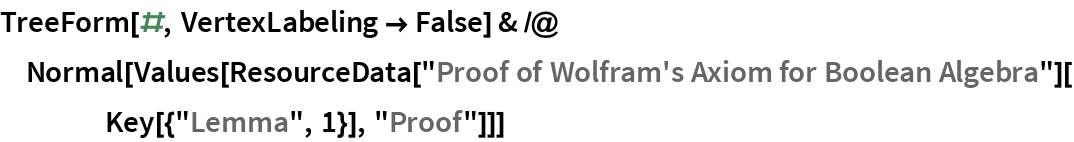Out=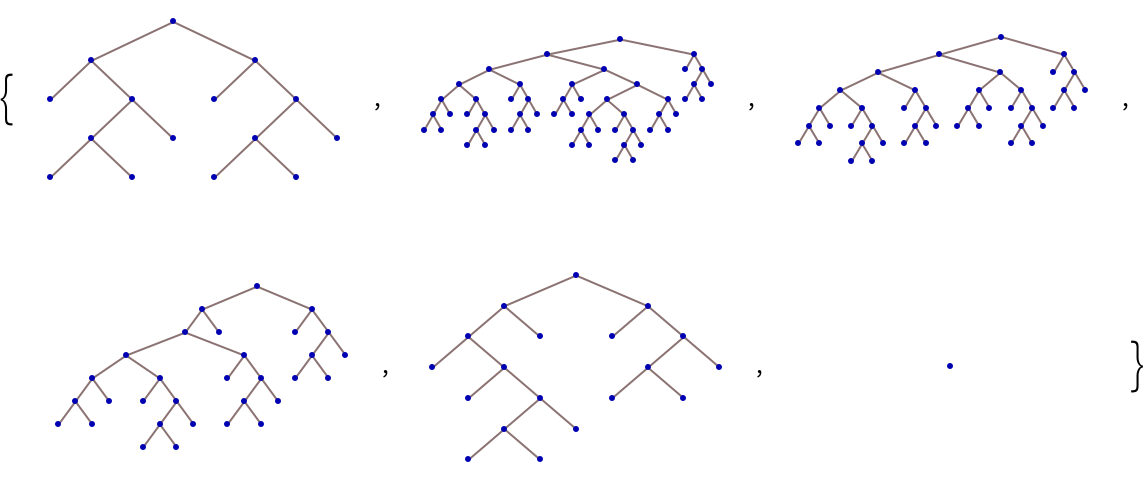#### Visual representations of the statements on the first 10 steps:

 In:=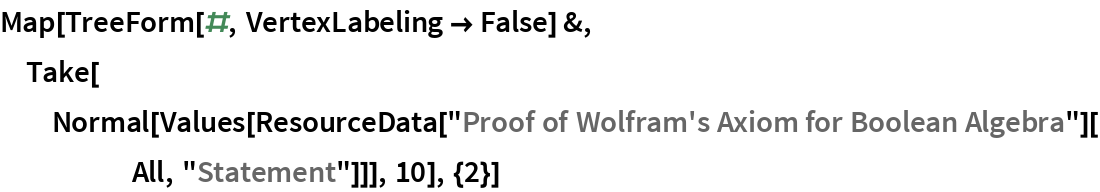Out=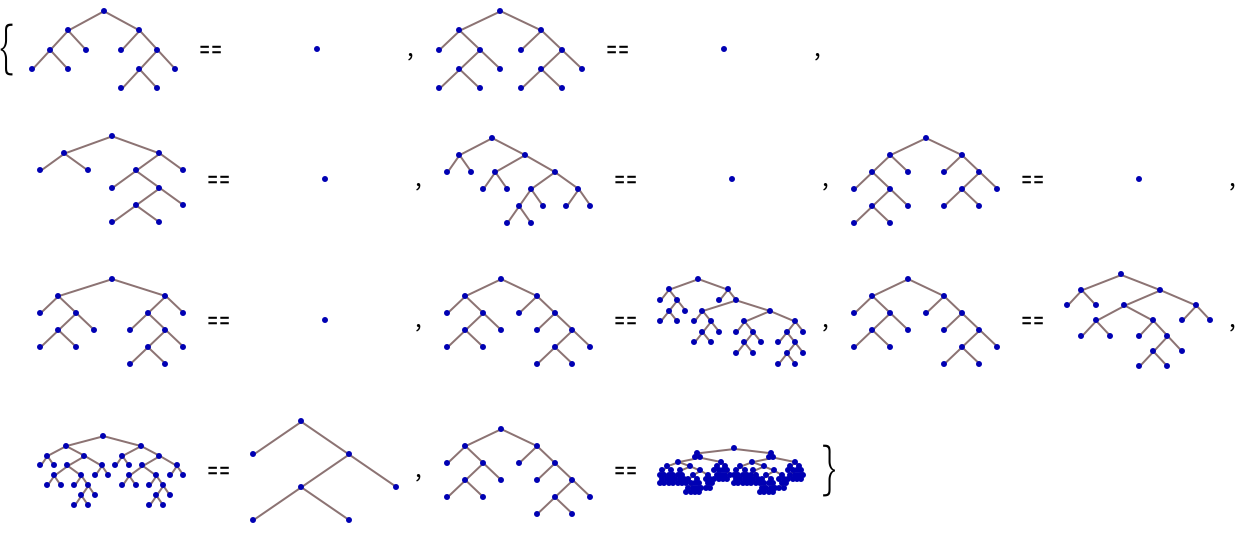In:=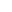### Analysis

Wolfram Research, "Proof of Wolfram's Axiom for Boolean Algebra" from the Wolfram Data Repository (2017)